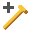Expert Modelling: Hands-on help from Rocscience Engineers. Register Here

# Support Overview

There are various ways of modeling support in RocPlane:

• Bolts can be added to the model with the Add Bolt option.
• An average support or loading pressure can be defined with the Pressure option.
• A force can be applied to the wedge with the External Force option.

It is important to remember that all of the methods of modeling support in RocPlane simply represent different ways of applying a force to the wedge. Like all other forces in the RocPlane analysis, support forces are applied through the centroid of the wedge, and are specified per unit width of slope.

The different support options allow you to choose whichever method is most convenient. For example:

## Bolts

• A bolt added with the Add Boltoption does not necessarily have to represent a single bolt, but could represent the total support force applied by all bolts in a pattern that have the same orientation. The magnitude of the bolt capacity can be entered as the total capacity of all bolts.

## External Force

• A support force can also simply be modeled as an External Force. An External Force is exactly equivalent to a bolt added with the Add Bolt option if the magnitude and orientation are the same and the bolt model is ACTIVE. See How Bolts Are Implemented in RocPlane for details.

## Pressure

• The Pressure option allows you to specify a support or loading pressure in terms of force per unit area of the wedge face (either the slope face or the upper slope). This is the easiest way to model the effect of pattern bolting, or surcharge loads, because the total force applied to the wedge is automatically calculated by RocPlane based on the wedge face area(s) and the pressure magnitude.
• In a Probabilistic analysis, if the joint orientations are random variables, the actual size and shape of the wedge changes with each simulation. In this case, the Pressure option is the recommended way to properly model support since it automatically accounts for the changing surface area of the wedge.